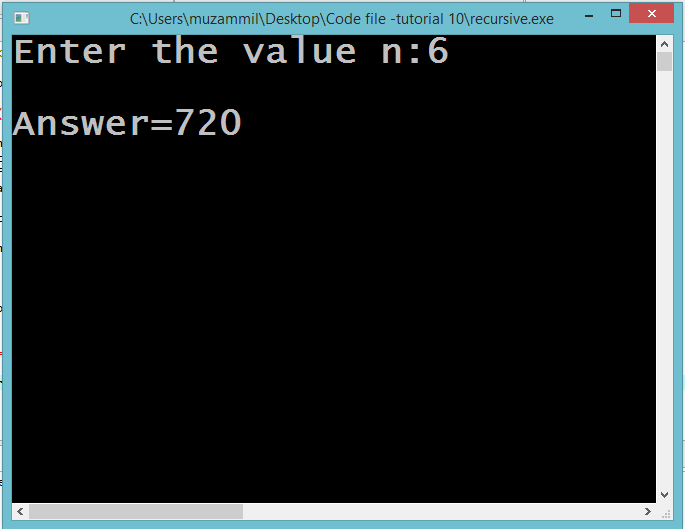In this part you will learn: 1. C syntax 2. Functions 3. Function Calling 4. Function definition 5. Function prototype 6. Recursive Functions 7. Showing output

### What is Recursion?

Recursion is a process of repeating of items in a self-similar way. A recursive function is a function that calls itself and this technique is known as recursion in C programming. Recursion helps to make functions precise and easy. Recursive Function Basic Step: Open Dev C++ then File > new > source file and start writing the code below.
`#include<stdio.h>#include<conio.h>`
These two are the most common and basic header files. The first one stdio.h is used to grant us most of the basic functions of C like Input and Output functions. The second one conio.h provides us functions like getch() which is used to pause our screen until we press a button.
`int factorial(int a); int main(){	int n;	printf("Enter the value n:");	scanf(" %d",&n);	int answer=factorial(n); 	printf("\nAnswer=%d",answer);; 	getch(); }`
In this program we are going to calculate the factorial of number that has been entered by the user using recursion. We will write a recursive function that will keep on calling itself until a certain condition is met. First of all we declared the function prototype. The function takes one parameter which is an integer and returns a integer. Then we started our main function. In this function we declared a integer variable and took input from the user in that variable. After that we passed that variable to the function. Note that when we pass a variable to a function it is passed by value. This means another copy of that variable is made in the memory with same value but different name. Similarly when a variable is declared in any function the scope of the variable is that function. This means that when the program exits that particular function that variable is deleted from the memory. The factorial function returns us the answer of the calculation and then we store that answer in a new variable. At the end we print the answer and pauses our program
`int factorial(int a){ 	if(a==0)	{		return 1;	} 	else		return a*factorial(a-1);  }`
Now there is our function definition. In the definition we first placed a condition that checks if our number is zero the function returns 1 as the factorial of zero is one. Otherwise the program goes in the else part. In the else part what we are doing is multiplying our number with number that will be return by our function when it is called for a value which is 1 less than our original number. So what happens is when we call this function for a particular number it calls itself for a number which is 1 less than the number. It keeps on doing this operation until the number in the variable ‘a’ becomes 0. When the value of the variable becomes 0 the function return 1. Now the function starts to return all those calls as many times as it was called from its own self. In this way this function computes the factorial of the number entered by the user. And at last returns the original answer to main function. Execute > compile then Execute > run Output